# NCERT Solutions For Class 8 Maths Chapter 9 Exercise 9.4

NCERT Solutions for Class 8 Maths Chapter 9 Exercise 9.4 – Algebraic Expressions & Identities, has been designed by the NCERT to test the knowledge of the student on the topic – Multiplying a Polynomial by a Polynomial

• Multiplying a binomial by a binomial
• Multiplying a binomial by a trinomial

### NCERT Solutions For Class 8 Maths Chapter 9 Exercise 9.4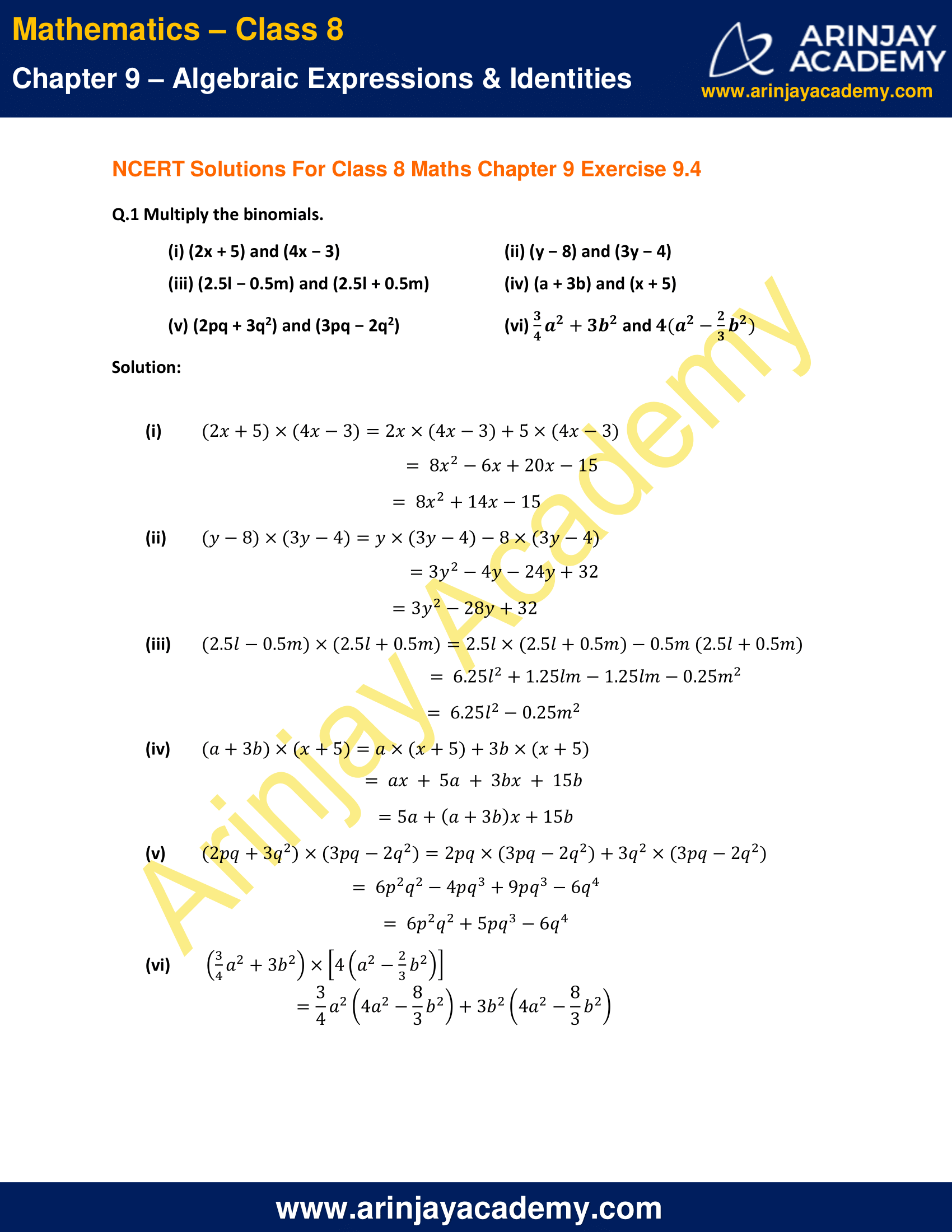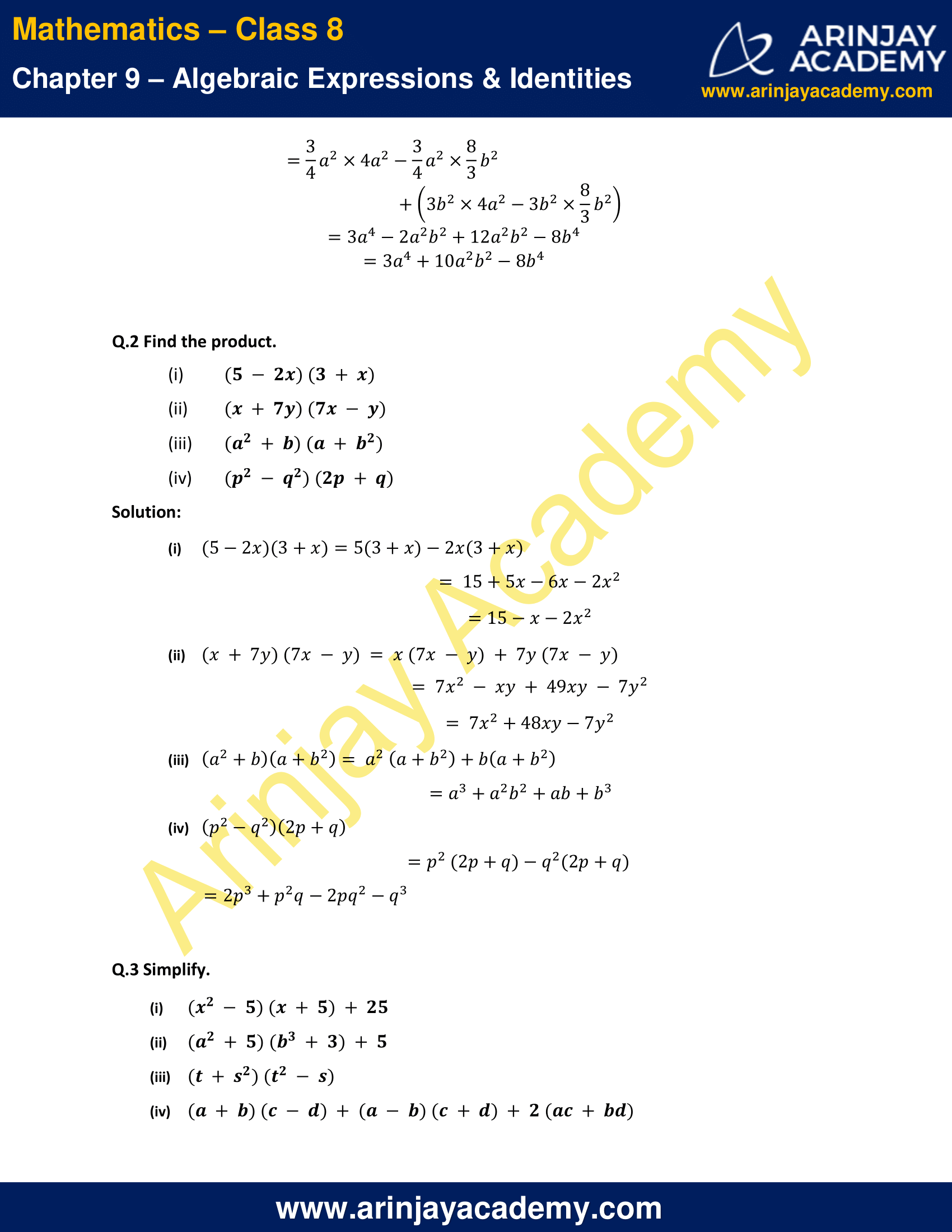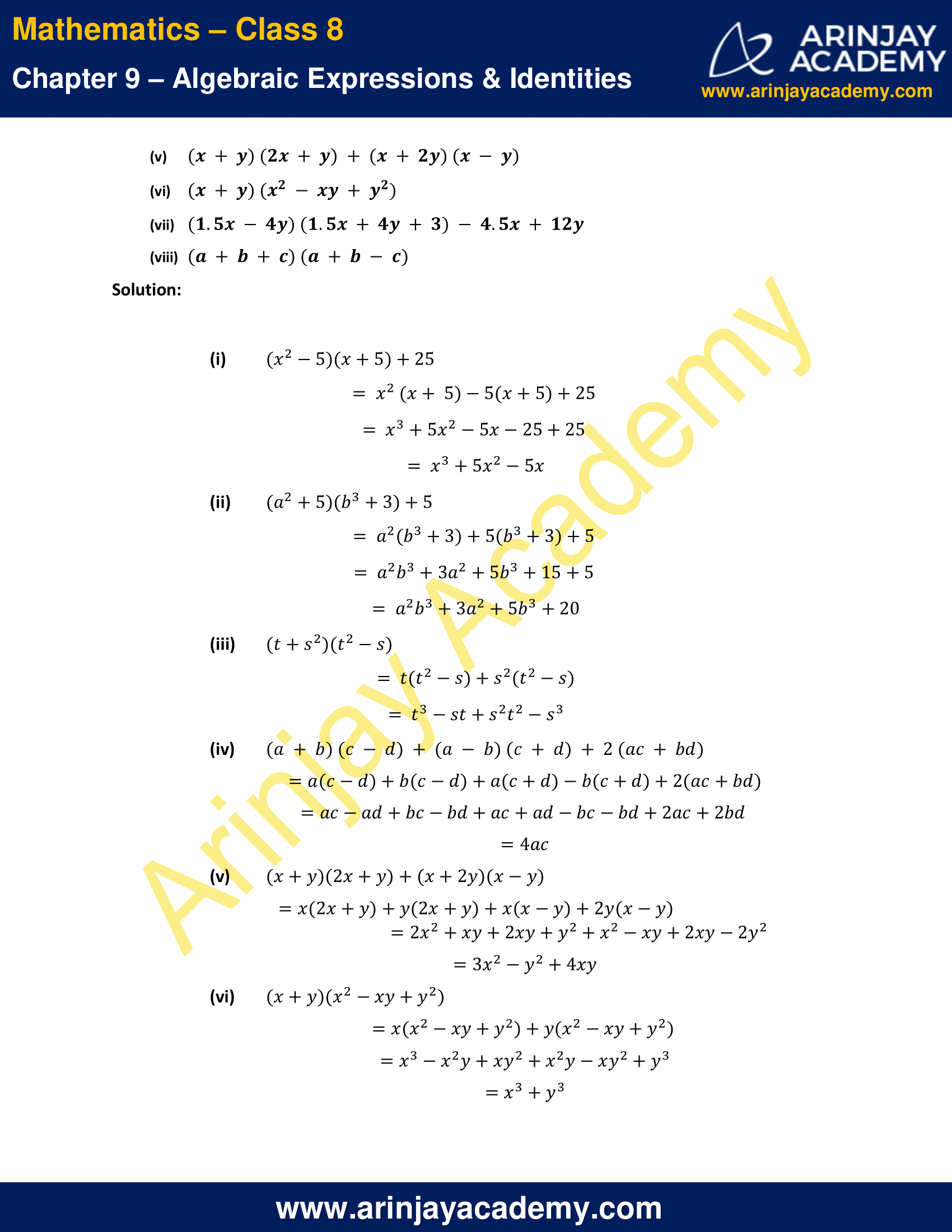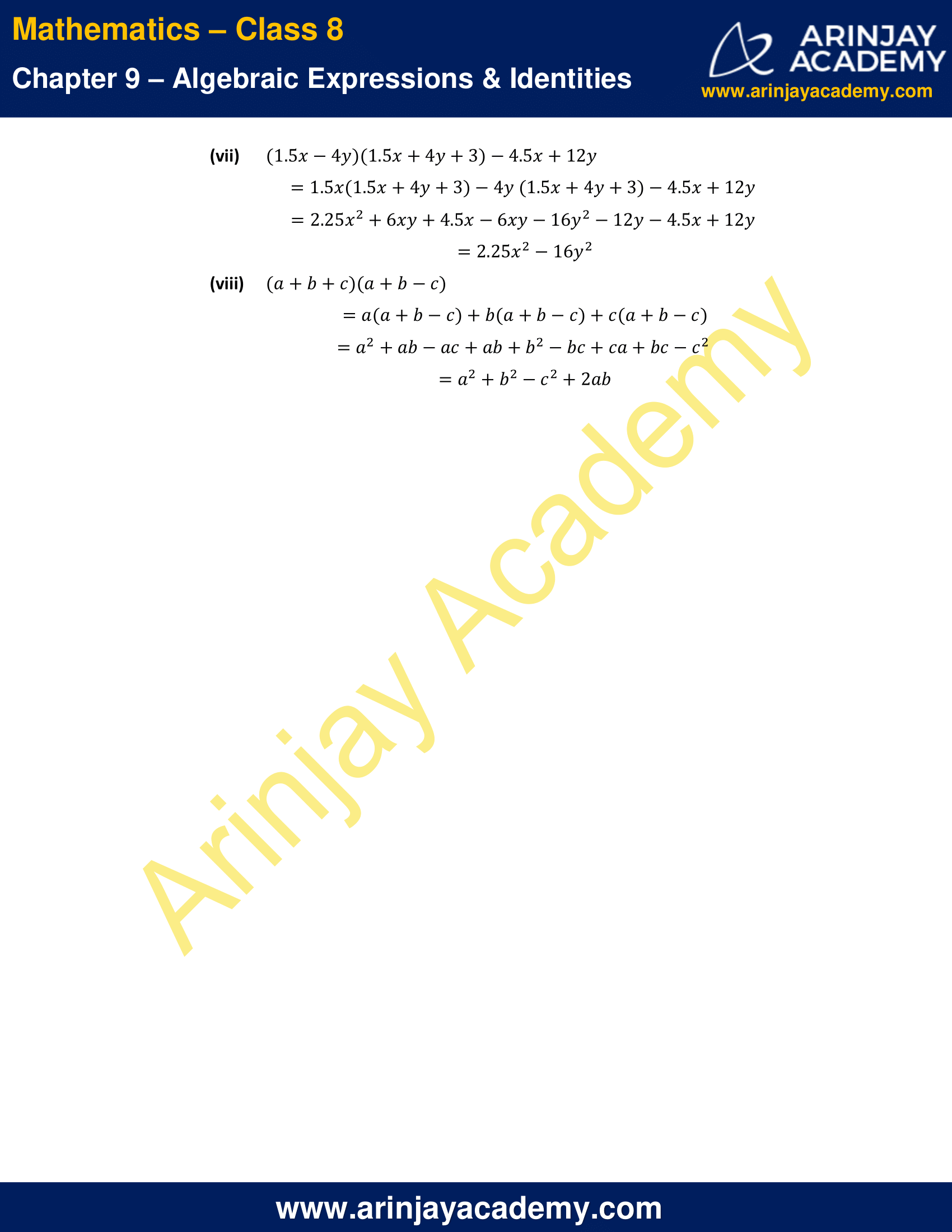NCERT Solutions For Class 8 Maths Chapter 9 Exercise 9.4

Q.1 Multiply the binomials.

(i)(2x+5)and(4x−3)
(ii)(y−8)and(3y−4)
(iii)(2.5l−0.5m)and(2.5l+0.5m)
(iv)(a+3b)and(x+5)
(v)(2pq+3q²)and(3pq−2q²)
(vi) (3/4)a² + 3b² and 4[a² – (2/3)b²]

Answer:

i. (2x+5) × (4x-3)
=2x × (4x-3)+5 × (4x-3)
=8x²-6x+20x-15
=8x²+14x-15

ii. (y-8) × (3y-4)
=y × (3y-4)-8 × (3y-4)
=3y²-4y-24y+32
=3y²-28y+32

iii. (2.5l-0.5m) × (2.5l+0.5m)
=2.5l × (2.5l+0.5m)-0.5m(2.5l+0.5m)
=6.25l²+1.25lm-1.25lm-0.25m²
=6.25l²-0.25m²

iv. (a+3b) × (x+5)
=a × (x+5)+3b × (x+5)
=ax+5a+3bx+15b
=5a+(a+3b)x+15b

v. (2pq+3q²) × (3pq-2q²)
=2pq × (3pq-2q²)+3q² × (3pq-2q²)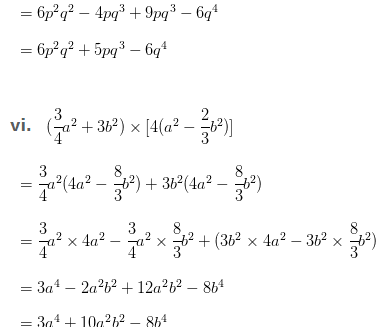Q.2 Find the product.

i. (5 – 2x) (3 + x)
ii. (x + 7y) (7xy)
iii. (a2 + b) (a + b2)
iv. (p2q2) (2p + q)

i. (5-2x)(3+x)=5(3+x)-2x(3+x)
= 15+5x-6x-2x2
=15-x-2x2

ii. (x + 7y) (7x – y)
= x (7x – y) + 7y (7x – y)
= 7x2 – xy + 49xy – 7y2
= 7x2+48xy-7y2

iii. (a2+b)(a+b2)
= a2 (a+b2)+b(a+b2)
=a3+a2b2+ab+b3

iv. (p2-q2)(2p+q)
=p2 (2p+q)-q2(2p+q)
=2p3+p2q-2pq2-q3

Q.3 Simplify.

i. (x2 – 5) (x + 5) + 25
ii. (a2 + 5) (b3 + 3) + 5
iii. (t + s2) (t2 – s)
iv. (a + b) (cd) + (ab) (c + d) + 2 (ac + bd)
v. (x + y) (2x + y) + (x + 2y) (xy)
vi. (x + y) (x2xy + y2)
vii. (1.5x – 4y) (1.5x + 4y + 3) – 4.5x + 12y
viii. (a + b + c) (a + bc)

Solution:

i. (x2-5)(x+5)+25
= x2 (x+ 5)-5(x+5)+25
= x3+5x2-5x-25+25
= x3+5x2-5x

ii. (a2+5)(b3+3)+5
= a2(b3+3)+5(b3+3)+5
= a2b3+3a2+5b3+15+5
= a2b3+3a2+5b3+20

iii. (t+s2)(t2-s)
= t(t2-s)+s2(t2-s)
= t3-st+s2t2-s3

iv. (a + b) (c – d) + (a – b) (c + d) + 2 (ac + bd)
=a(c-d)+b(c-d)+a(c+d)-b(c+d)+2(ac+bd)
=ac-ad+bc-bd+ac+ad-bc-bd+2ac+2bd
=4ac

v. (x+y)(2x+y)+(x+2y)(x-y)
=x(2x+y)+y(2x+y)+x(x-y)+2y(x-y)
=2x²+xy+2xy+y²+x²-xy+2xy-2y²
=3x²-y²+4xy

vi. (x+y)(x2-xy+y2)
=x(x²-xy+y²)+y(x²-xy+y²)
=x³-x²y+xy²+x²y-xy²+y³
=x³+y³

vii. (1.5x-4y)(1.5x+4y+3)-4.5x+12y
=1.5x(1.5x+4y+3)-4y (1.5x+4y+3)-4.5x+12y
=2.25x²+6xy+4.5x-6xy-16y²-12y-4.5x+12y
=2.25x²-16y²

viii. (a+b+c)(a+b-c)
=a(a+b-c)+b(a+b-c)+c(a+b-c)
=a²+ab-ac+ab+b²-bc+ca+bc-c²
=a²+b²-c²+2ab

The next Exercise for NCERT Solutions for Class 8 Maths Chapter 9 Exercise 9.5 – Algebraic Expressions and Identities can be accessed by clicking here

NCERT Solutions For Class 8 Maths Chapter 9 Exercise 9.4 – Algebraic Expressions & Identities